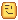# How should I understand torque, angular velocity etc

I couldn’t understand what a Torque is I searched for hours online trying to figure out what torque then I found it is something to understand in 11 th grade physics. But I’m in 8th grade. So am I able to learn it??

Torque is the force required to put an angular force on something (rotate it).
When you tighten a nut you apply torque.
When you turn a wheel you apply torque.
When you spin a Part around by one of its edges you are applying torque to that location on the Part.

Check out MotorMaxTorque in HingeConstraints.

Torque and speed are proportionally related, if your motor generates 5 ft-lb of torque and has an angular velocity of 20rpm, then a reduction of 10:1 will cause your output shaft to have 50 ft-lb of torque with an angular velocity of 2rpm. Now gears aren’t a thing in Roblox but it goes to show how having a high torque tends to have a smaller velocity, but don’t get me wrong you could have high torque and high angular velocity. In Roblox, it is super simple you just set the angular velocity for your motor and it goes, that’s why it tends to be useless to have a realistic torque in Roblox and math.huge is preferable.You can learn more about torque and angular velocity from this POE - Gear Ratios Calculations - YouTube

I should note that your rpm will be slowed down based on how much load is in your system, like if you had a car with 600 rpm but 500 ft-lb of torque and your car weighed 250 lbs then your rpm would be roughly 300, it will be exactly 300 in roblox however

Actually Torque plays a factor in the way vehicles handle similar to real life. Torque actually afffects acceleration, not output rpm.

How does high Torque on a Roblox wheel give low velocity? AngularVelocity is the rpm of the HingeConstraint. Like in real life where the gear ratio and engine/motor rpm determine the rpm of the output, the amount of torque on the HingeConstraint + the AngularVelocity determines the rpm of the output.
The forward speed of the vehicle depends on the AngularVelocity of the HIngeConstraint attached to the wheel and the circumference of the wheel (the measurement around the wheel).

A HingeConstraint has MotorMaxTorque, MotorMaxAcceleration and AngularVelocity as its Properties. The acceleration limits how quickly a motor gets to its AngularVelocity value.

If you have low torque on a large wheel it may not move.
If you have high torque on a vehicle that runs on a lower friction then it’ll start to spin the wheels unless you lower the MotorMaxAcceleration so it’s not ‘full throttle’ right at the start.

I thought it affected rpm as Tout/Tin = Win/Wout. I never stated that high torque on a Roblox wheel gives low velocity and in fact, I actually stated that it doesn’t matter on Roblox as it automatically scales the system so you usually just set the max torque to math.huge. I’m probably missing something here, but rpm is a form of angular velocity but torque isn’t from my understanding. How would adding them give you the rpm when your rpm is simply `(AngularSpeed/6.28) / 60`? The only reason why the forward speed of the vehicle is dependent on the circumference of the wheel is cause you are covering more area per revolution.

Looks like you edited your first post.I should have put “&” instead of “+” in that sentence, it was never meant to be a formula.

What I meant was that even though rpm is a measurement of AngularVelocity in a HingeConstraint, because there are so many other variables such as vehicle mass, wheel mass, if the Torque is less than math.huge, MotorMaxAcceleration etc. sometimes you don’t get the results you’d expect. For example just because you make the AngularVelocity 25 radians/sec in a HingeConstraint doesn’t mean it will necessarily reach that value.

I have used less than math.huge MotorMaxTorque in many of my projects to fine tune how they react in different situations.

I was trying to explain to a grade 8 student a simplified version of torque and angular velocity without getting into the math involved so they could understand how changing any of the Properties of a HingeConstraint will affect how it reacts.

1 Like